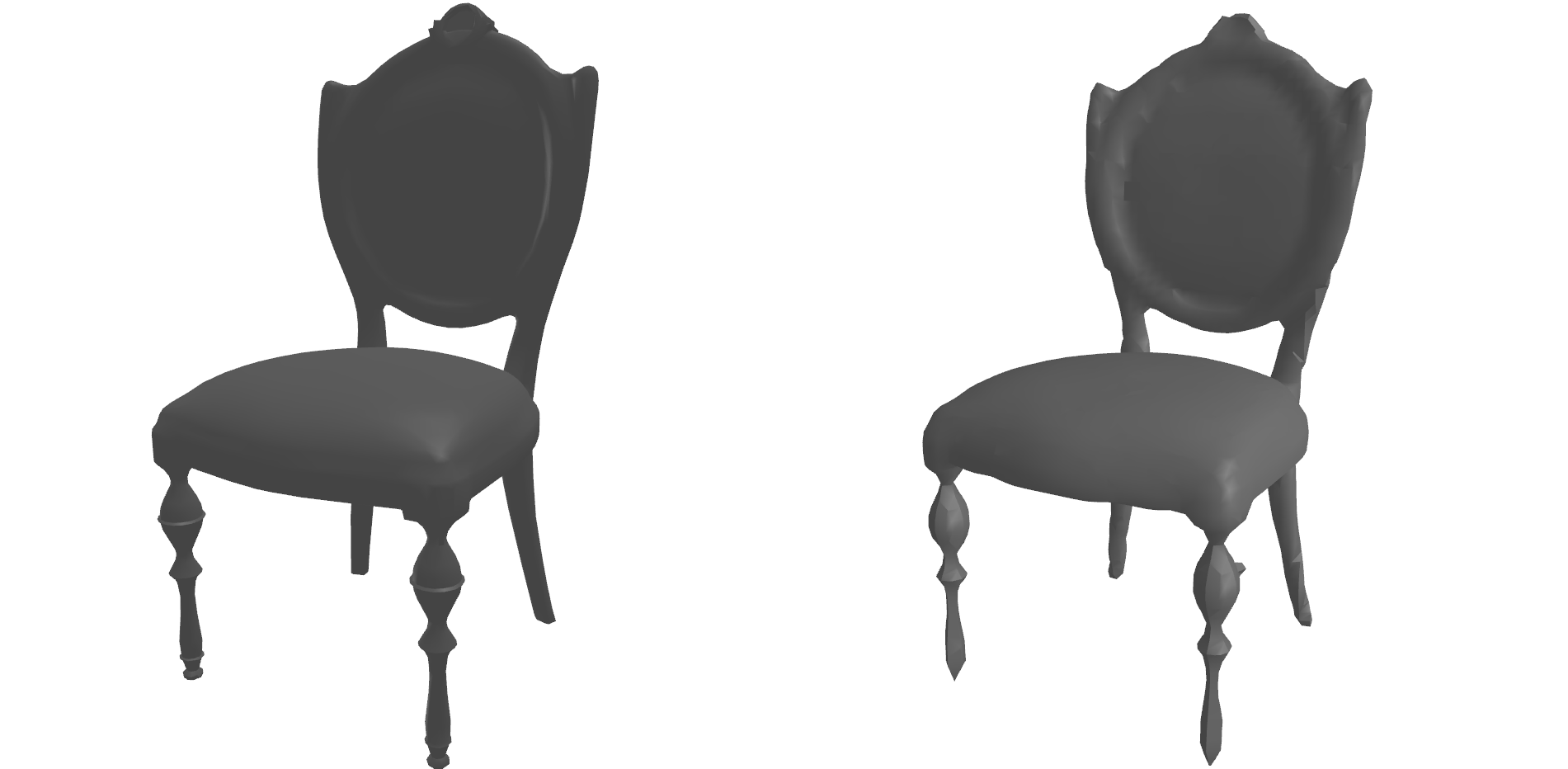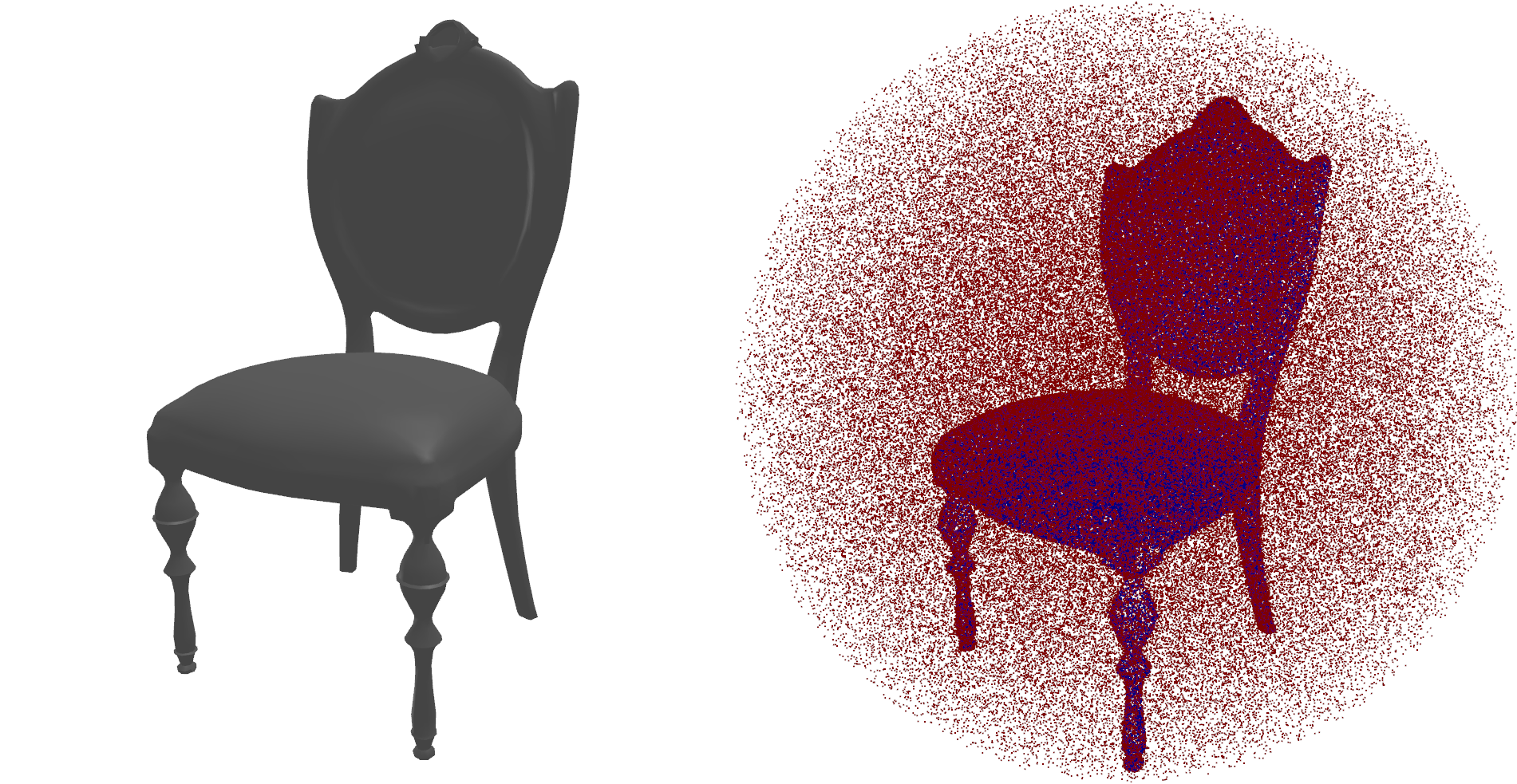Showing:

0

392

6mos ago

3

4

# Calculate signed distance fields for arbitrary meshes

This project calculates approximate SDFs for triangle meshes. It works for non-watertight meshes (meshes with holes), self-intersecting meshes, meshes with non-manifold geometry and meshes with inconsistently oriented faces.

## Install

``````pip3 install mesh-to-sdf
``````

If you're using Windows or Mac, you need to work around a bug in pyrender. Check the FAQs below.

## Examples

### Voxelize a mesh

The `mesh_to_voxels` function creates an N ✕ N ✕ N array of SDF values. In this example, a mesh is reconstructed using Marching Cubes and then rendered.

``````from mesh_to_sdf import mesh_to_voxels

import trimesh
import skimage

vertices, faces, normals, _ = skimage.measure.marching_cubes(voxels, level=0)
mesh = trimesh.Trimesh(vertices=vertices, faces=faces, vertex_normals=normals)
mesh.show()
``````### Sample SDF points non-uniformly near the surface

This example creates 250,000 points, where most of the points are close to the surface and some are sampled uniformly. This is the method that is proposed and used in the DeepSDF paper. In this example, the resulting points are rendered in red where the SDF is positive and in blue where it is negative.

``````from mesh_to_sdf import sample_sdf_near_surface

import trimesh
import pyrender
import numpy as np

points, sdf = sample_sdf_near_surface(mesh, number_of_points=250000)

colors = np.zeros(points.shape)
colors[sdf < 0, 2] = 1
colors[sdf > 0, 0] = 1
cloud = pyrender.Mesh.from_points(points, colors=colors)
scene = pyrender.Scene()
viewer = pyrender.Viewer(scene, use_raymond_lighting=True, point_size=2)
``````## How it works

The general pipeline for calculating SDF in this project is as follows:

1. Create 100 virtual laser scans of the shape from multiple angles. These each consist of a normal buffer and a depth buffer.
2. Use the inverse MVP matrix and depth buffer of each scan to calculate a world-space surface point cloud
3. Determine the value of the SDF for each query point by finding the closest surface point using a kd-tree
4. Determine the sign of the SDF using either the normal of the closest surface point or by checking it against the depth buffers of the scans. When using normals, the sign is determined with a dot product. When using the depth buffer method, the point is projected in the frame of each render. By comparing the depth element of the depth buffer and depth of the query point, we determine if the query point is seen by the camera. The sign of the point is positive if it is seen by any of the cameras.

This repository contains an implementation of the procedure proposed in the DeepSDF paper, as well as some alternatives.

## FAQ

Q: I'm getting this error: `module 'pyglet.gl' has no attribute 'xlib'`

This is due to a bug in pyrender. Possible workarounds:

• use `pyrender 0.1.30` and `pyglet 1.4.0b1` (check this issue for more details)
• manually edit `site-packages/pyrender/platforms/pyglet_platfform.py` and surround the content of `make_uncurrent` with a try-catch block
• use Linux instead of Windows or Mac. This problem appears because pyrender tries to use a library that is only available on Linux.

Q: I want to run this on a computer without a screen (ie. via SSH)

Add this to your script before importing `mesh_to_sdf`:

``````import os
os.environ['PYOPENGL_PLATFORM'] = 'egl'
``````

Q: There are cone shaped artifacts in the SDF volume when using `sign_method='normal'`

This is a known issue. To mitigate this, the signs are determined by checking a number of surface points and using a majority vote. This problem can't be avoided entirely and increasing the number of points (`normal_sample_count`) even further doesn't seem to help. In some cases, this problem appears when the mesh contains tiny triangles that face in a different direction than their surrounding area. Smoothing the mesh doesn't seem to help.

The `sign_method='depth'` approach doesn't have this problem. But it doesn't work with meshes that have holes.

Q: There are ray shaped artifacts in the SDF volume when using `sign_method='depth'`

This happens when the mesh has holes and a camera can see "inside" the mesh. Use `sign_method='normal'` instead.

Q: This doesn't work!

This repository contains two approximate methods and in some cases they don't provide usable results. When one of the methods fails, try the other one. This can be automated by using the built-in ways to check if the result is plausible. For the voxelizing methods, use `check_result=True`. This checks if the difference in SDF is smaller than the difference in distance between any two points. In `sample_sdf_near_surface`, you can add a volume check (`min_size`). If these checks fail, a `BadMeshException` is thrown.

This method can process about 60% of the meshes in the ShapeNet dataset.

# Documentation

## mesh_to_sdf

Calculate signed distance values for an array of given query points

``````mesh_to_sdf.mesh_to_sdf(mesh, query_points, surface_point_method='scan', sign_method='normal', bounding_radius=None, scan_count=100, scan_resolution=400, sample_point_count=10000000, normal_sample_count=11)
``````

Parameters

• `mesh`: a trimesh mesh
• `query_points`: an N ✕ 3 numpy array, containing the points for which the signed distance function should be calculated.
• See common parameters for the remaining parameters

Returns

• a numpy array with N elemenents, containing the SDF for each query point

## mesh_to_voxels

Calculate an N ✕ N ✕ N voxel volume of signed distance values for a given mesh. The mesh is first transformed to fit inside a cube ranging from -1 to 1.

``````mesh_to_sdf.mesh_to_voxels(mesh, voxel_resolution=64, surface_point_method='scan', sign_method='normal', scan_count=100, scan_resolution=400, sample_point_count=10000000, normal_sample_count=11, pad=False, check_result=False, return_gradients=False)
``````

Parameters

• `mesh`: a trimesh mesh
• `voxel_resolution`: the resolution N of the resulting voxel volume
• `pad`: if `True`, the resulting array is padded with ones, ensuring a mesh without holes when reconstructing with Marching Cubes
• `check_result`: if `True`, the result is checked for continuity. If the voxel volume is not a plausible signed distance field, an exception is thrown.
• See common parameters for the remaining parameters

Returns

• a numpy array of shape (N, N, N) or (N + 2, N + 2, N + 2) when using padding

Returns additionally if `return_gradients` is `True`:

• gradients in a numpy array of shape (N, N, N, 3) or (N + 2, N + 2, N + 2, 3) when using padding

## sample_sdf_near_surface

Sample some points uniformly and some points near the shape surface and calculate SDFs for these points. This follows the procedure proposed in the DeepSDF paper. The mesh is first transformed to fit inside the unit sphere.

``````mesh_to_sdf.sample_sdf_near_surface(mesh, number_of_points = 500000, surface_point_method='scan', sign_method='normal', scan_count=100, scan_resolution=400, sample_point_count=10000000, normal_sample_count=11, min_size=0, return_gradients=False)
``````

Parameters

• `mesh`: a trimesh mesh
• `number_of_points`: the number N of points to be sampled, including surface points and uniform points
• `min_size`: The fraction of uniformly sampled that should be inside the shape. If this is 0.015 and less than 1.5% of uniformly sampled points have negative SDFs, an exception is thrown. This can be used to detect bad meshes.
• See common parameters for the remaining parameters

Returns

• an (N, 3) numpy array containing the sample points
• a numpy array of size N with the corresponding SDF values

Returns additionally if `return_gradients` is `True`:

• an (N, 3) numpy array containing the gradients

## get_surface_point_cloud

Returns an intermediate data structure containing a surface point cloud, scans and a kd-tree of the point cloud. This can be used if SDFs will be calculated multiple times for the same mesh or for debugging.

``````mesh_to_sdf.get_surface_point_cloud(mesh, surface_point_method='scan', bounding_radius=1, scan_count=100, scan_resolution=400, sample_point_count=10000000, calculate_normals=True)
``````

Parameters

• `mesh`: a trimesh mesh
• See common parameters for the remaining parameters

Returns

• an instance of `SurfacePointCloud`

## Common parameters

• `surface_point_method`: The method to generate a surface point cloud. Either `'scan'` or `'sample'`. The scanning method creates virtual scans while the sampling method uses the triangles to sample surface points. The sampling method only works with watertight meshes with correct face normals, but avoids some of the artifacts that the scanning method creates.

• `sign_method`: The method to determine the signs of the SDF values. Either `'normal'` or `'depth'`. The normal method uses normals of the point cloud. It works better for meshes with holes, but sometimes results in "bubble" artifacts. The depth method avoids the bubble artifacts but is less accurate.

• `bounding_radius`: The radius of a sphere that contains all mesh vertices. If `None`, this value is calculated using the mesh.

• `scan_count`: Number of scans when using the scanning method

• `scan_resolution`: Resolution for the scans in pixels.

• `sample_point_count`: Number of points to sample when using `surface_point_method='sample'`

• `normal_sample_count`: Number of nearby surface points to check when using `sign_method='normal'`. The sign of the resulting SDF is determined by majority vote.

## Rate & Review

Great Documentation0
Easy to Use0
Performant0
Highly Customizable0
Bleeding Edge0
Responsive Maintainers0
Poor Documentation0
Hard to Use0
Slow0
Buggy0
Abandoned0
Unwelcoming Community0
100